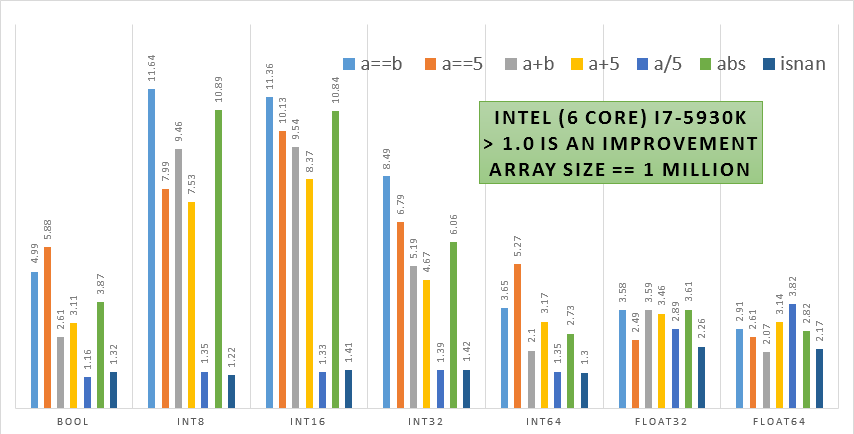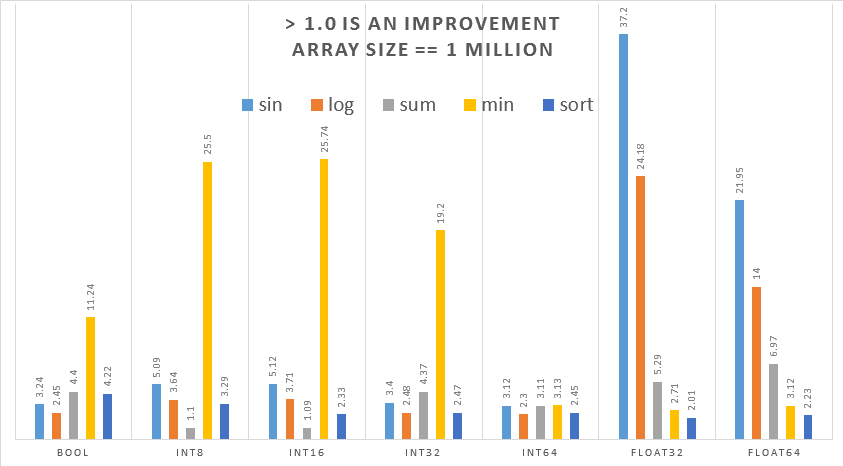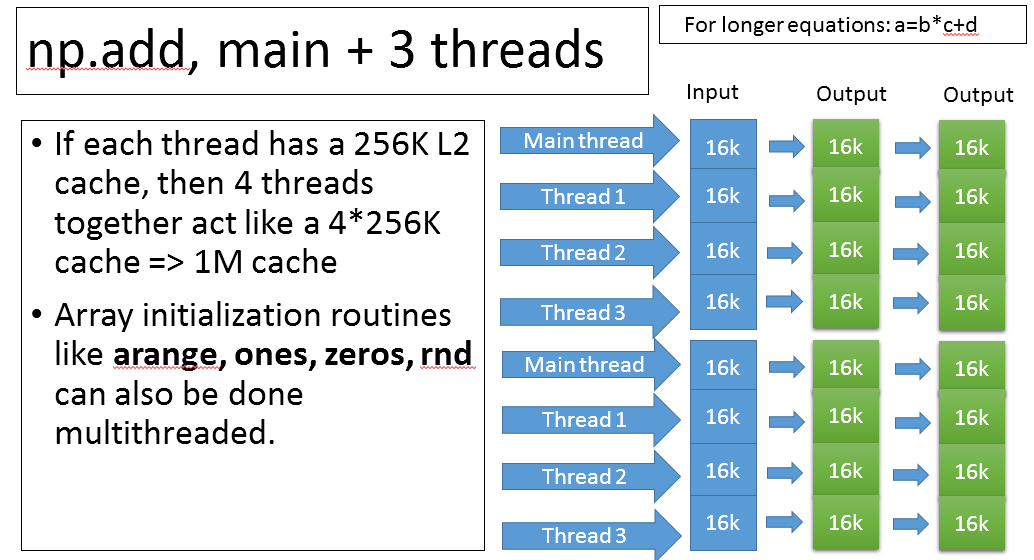# Welcome to PNumPy’s documentation!¶

PNumPy seamlessly speeds up NumPy for large arrays (64K+ elements) with no change required to your existing NumPy code.

This first release speeds up NumPy binary and unary ufuncs such as `add`, `multiply`, `isnan`, `abs`, `sin`, `log`, `sum`, `min` and many more. Sped up functions also include: `sort`, `argsort`, `lexsort`, boolean indexing, and fancy indexing. In the near future we will speed up: `astype`, `where`, `putmask`, `arange`, `searchsorted`.

## Installation¶

```pip install pnumpy
```

To use the project:

```import pnumpy as pn
```

PNumPy speeds up NumPy silently under the hood. To see some benchmarks yourself run ASV or use the built-in benchmark function:

```pn.benchmark()
```To get a partial list of functions sped up run

```pn.atop_info()
```

To disable or enable pnumpy run

```pn.disable()
pn.enable()
```

```pn.thread_setworkers(3)
```

PNumPy provides additional routines such as converting a NumPy record array to a column major array in parallel (pn.recarray_to_colmajor) which is useful for DataFrames. Other routines include pn.lexsort32, which performs an indirect sort using np.int32 instead of np.int64 consuming half the memory and running faster.

PNumPy uses a combination of threads and 256 bit vector intrinsics to speed up calculations. By default most operations will only use 3 additional worker threads in combination with the main python thread for a total 4. Large arrays are divided up into 16K chunks and threads are assigned to maintain cache coherency. More threads are dynamically deployed for more intensive CPU problems like np.sin. Users can customize threading. The example below shows how 4 threads can work together to quadruple the effective L2 cache size.## FAQ¶

Q: If I type np.sort(a) where a is an array, will it be sped up?

A: If len(a) > 65536 and pnumpy has been imported, it will automatically be sped up

Q: How is sort sped up?

A: PNumPy uses additional threads to divide up the sorting job. For example it might perform an 8 way quicksort followed by a 4 way mergesort

## Development¶

To run all the tests run:

```python -m pip install pytest
python -m pytest tests
```